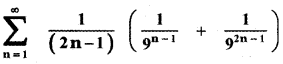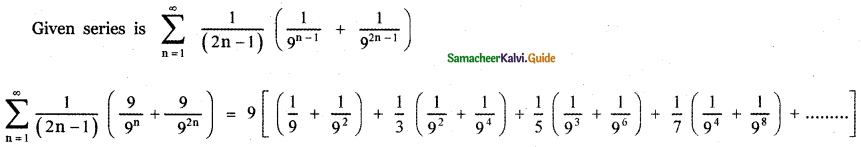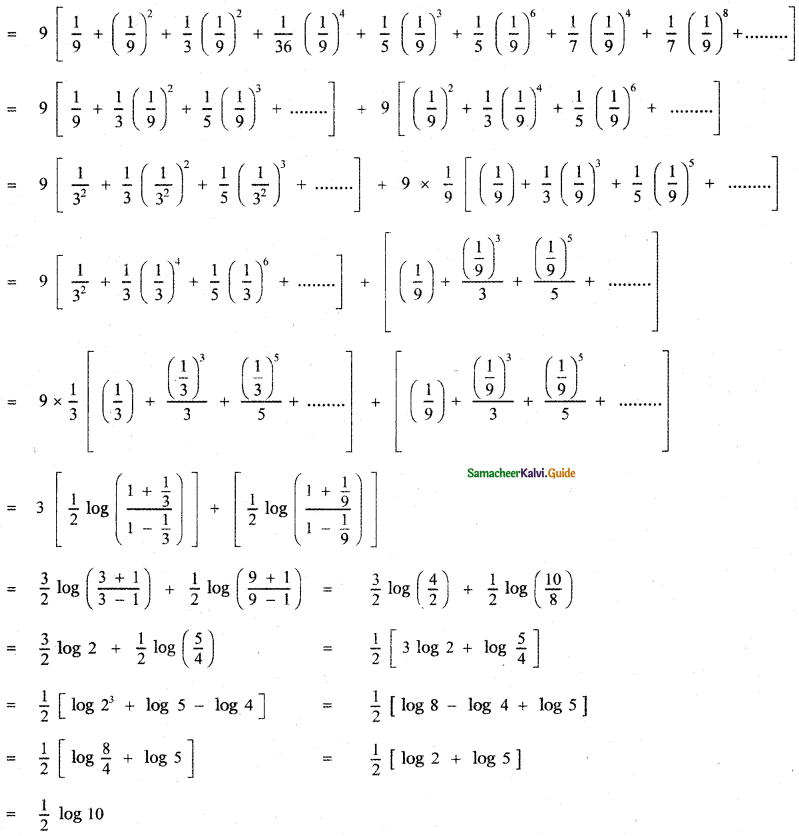Tamilnadu State Board New Syllabus Samacheer Kalvi 11th Maths Guide Pdf Chapter 5 Binomial Theorem, Sequences and Series Ex 5.4 Text Book Back Questions and Answers, Notes.

## Tamilnadu Samacheer Kalvi 11th Maths Solutions Chapter 5 Binomial Theorem, Sequences and Series Ex 5.4

Question 1.
Expand the following in ascending powers of x and find the condition on x for which the binomial expansion is valid.
(i) $$\frac{1}{5+x}$$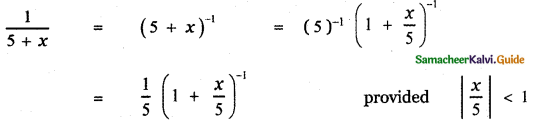(ii) $$\frac{2}{(3+4 x)^{2}}$$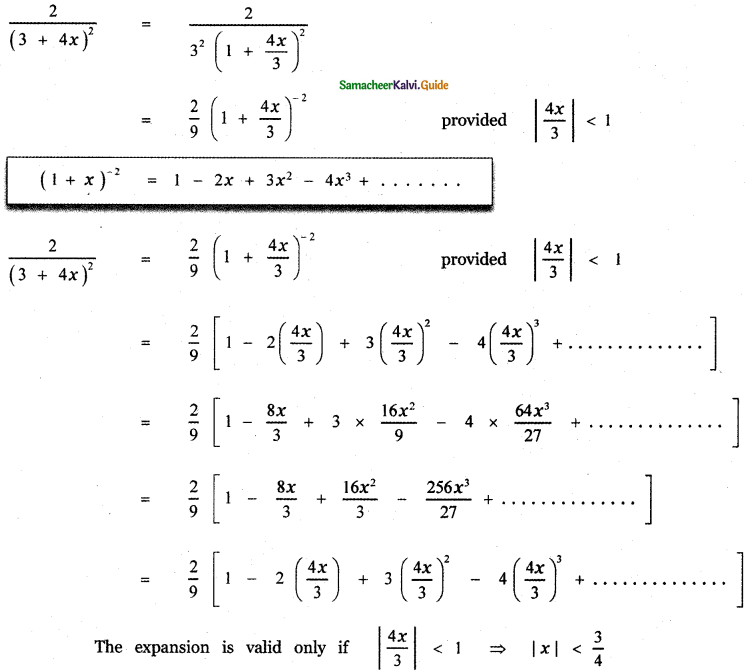(iii) (5 + x2)2/3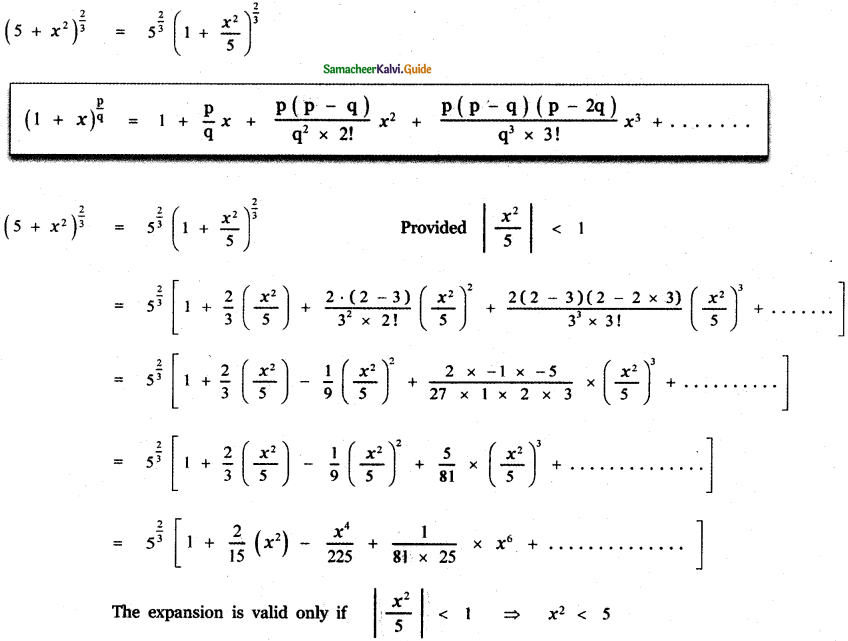(iv) $$(x+2)^{-\frac{2}{3}}$$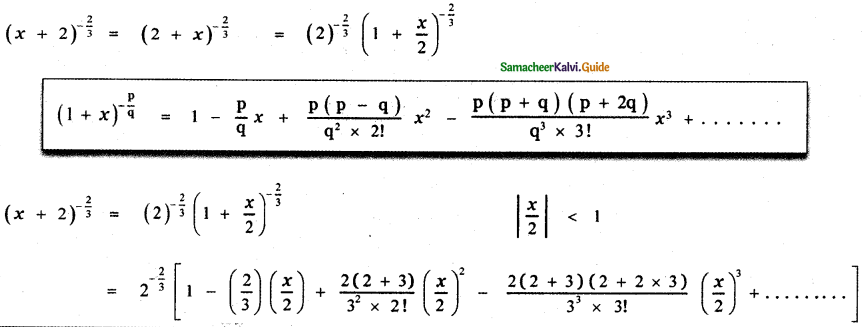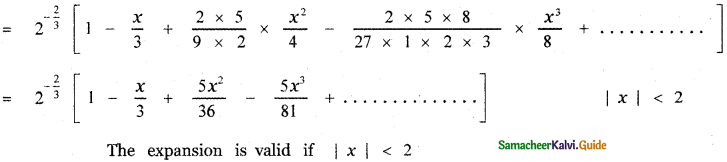Question 2.
Find $$\sqrt{1001}$$ approximately. (two decimal places)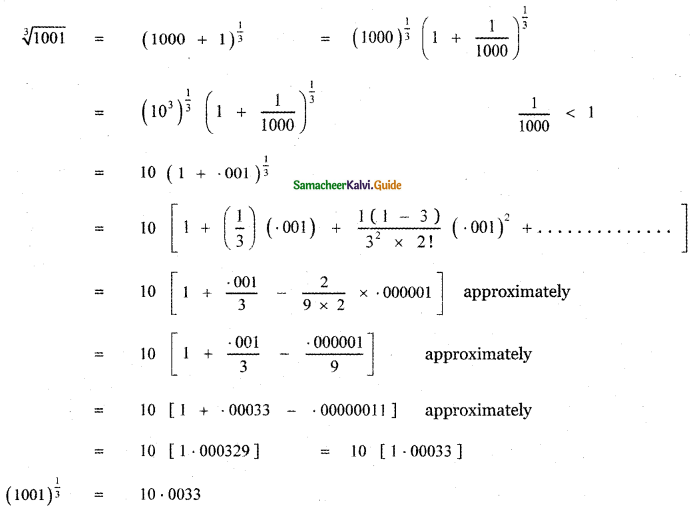Question 3.
Prove that $$\sqrt{x^{3}+6}-\sqrt{x^{3}+3}$$ is approximately equal to $$\frac{1}{x^{2}}$$ when x is sufficiently large.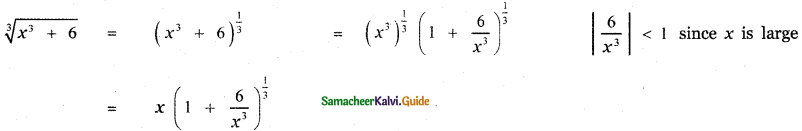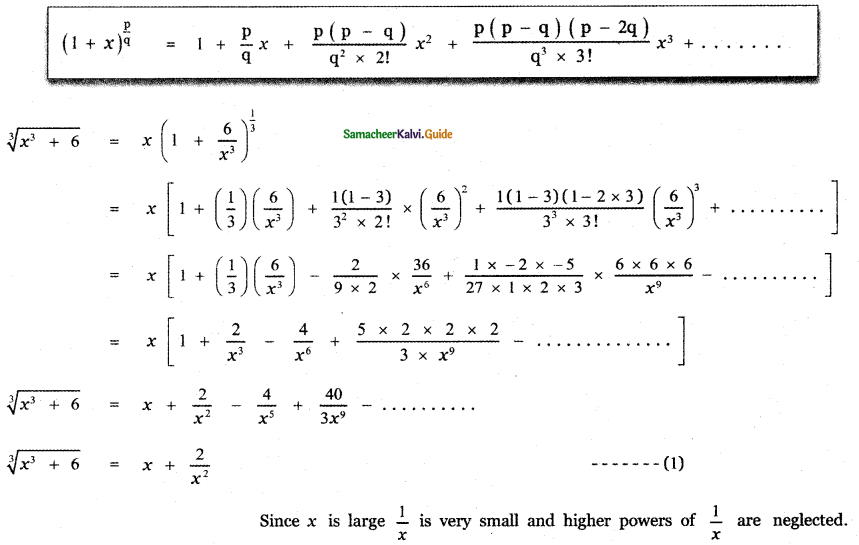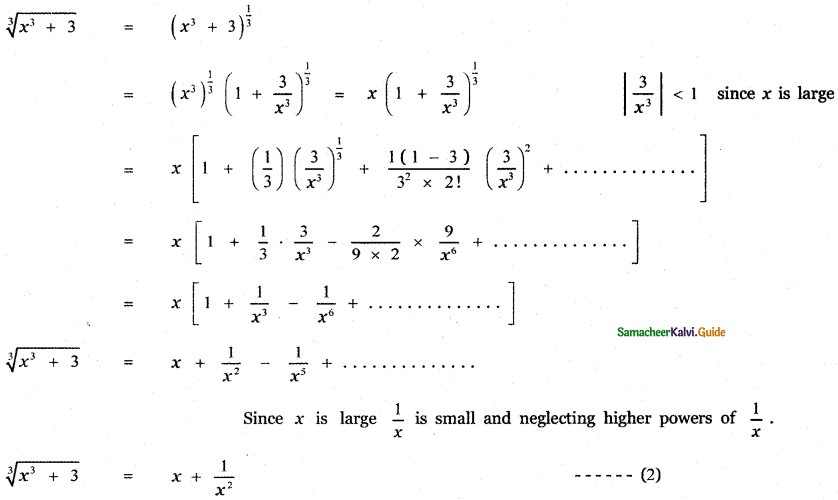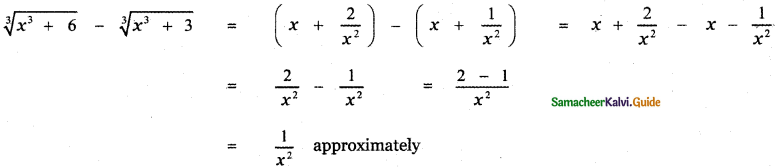Question 4.
Prove that $$\sqrt{\frac{1-x}{1+x}}$$ is approximately equal to 1 – x + $$\frac{x^{2}}{2}$$ when x is very small.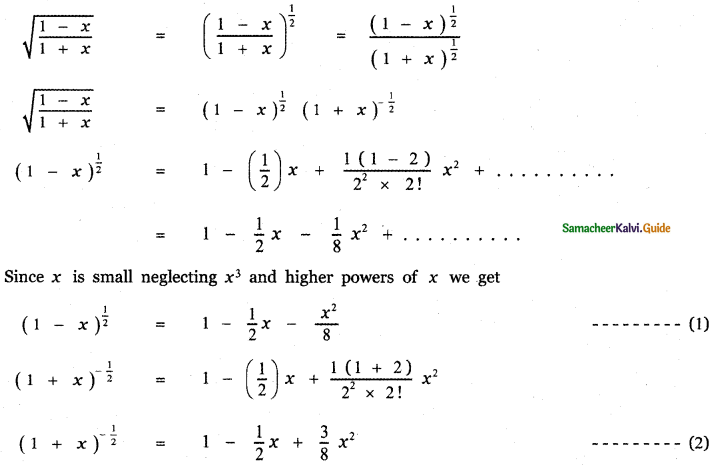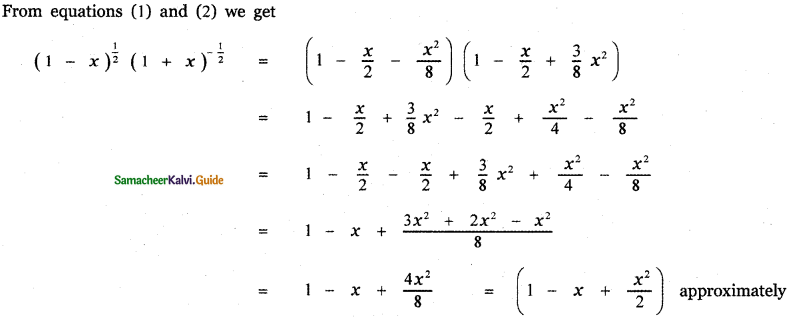Question 5.
Write the first 6 terms of the exponential series
(i) e5x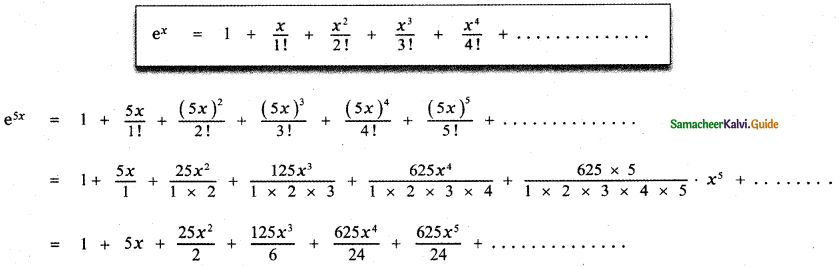(ii) e-2x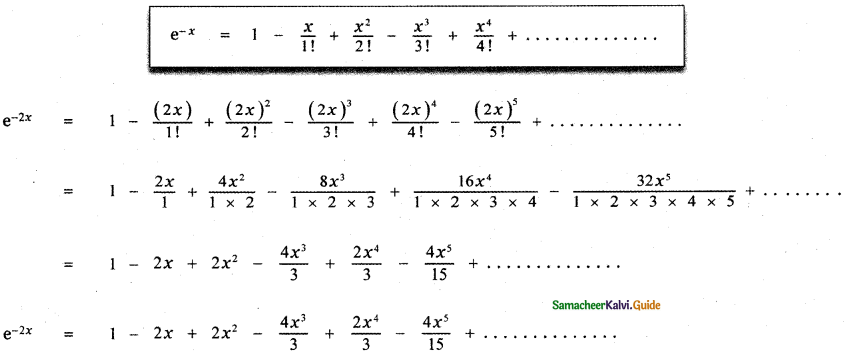(iii) ex/2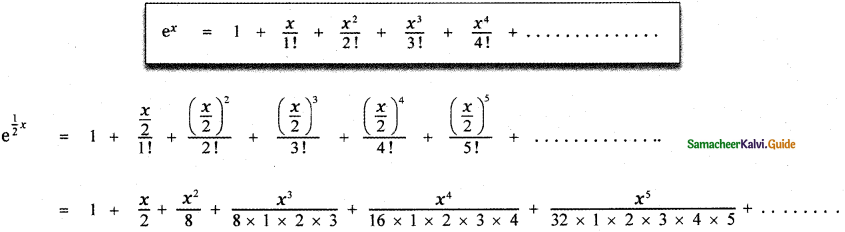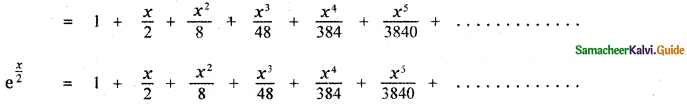Question 6.
Write the first 4 terms of the logarithmic series.
(i) log (1 + 4x)
(ii) log (1 – 2x)
(iii) log $$\left(\frac{1+3 x}{1-3 x}\right)$$
(iv) log $$\left(\frac{1-2 x}{1+2 x}\right)$$
Find the intervals on which the expansions are valid.
(i) log ( 1 + 4x )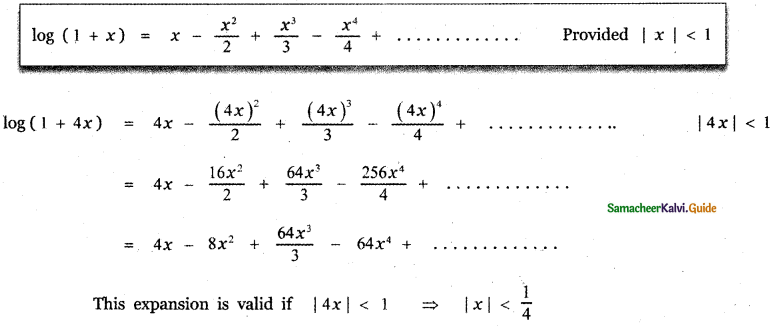(ii) log (1 – 2x)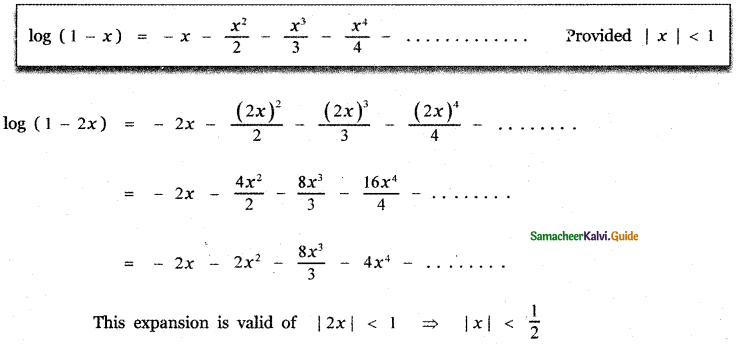(iii) log $$\left(\frac{1+3 x}{1-3 x}\right)$$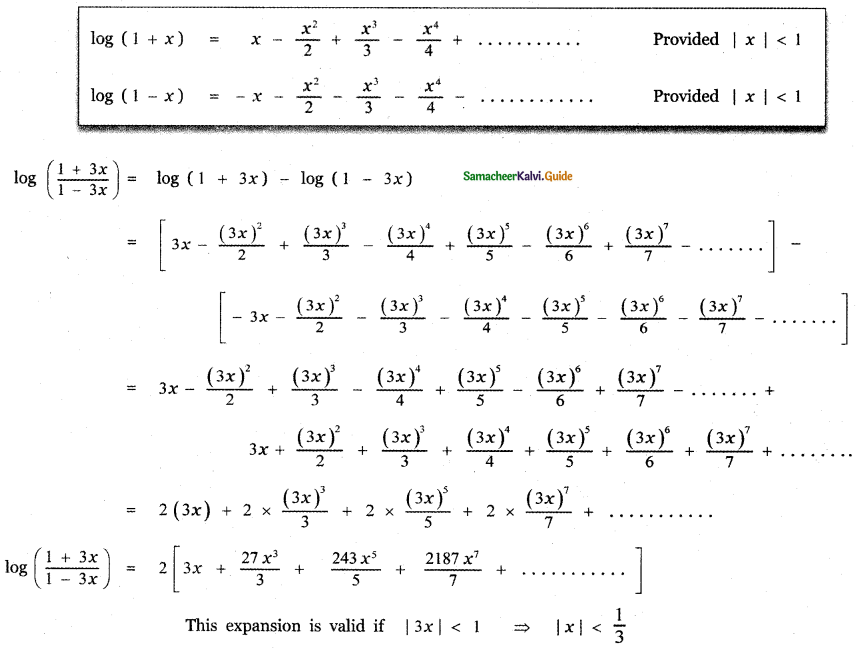(iv) log $$\left(\frac{1-2 x}{1+2 x}\right)$$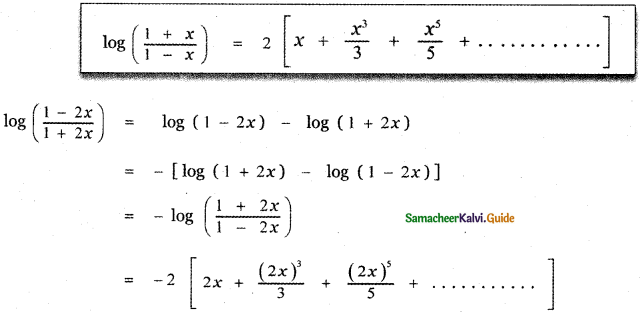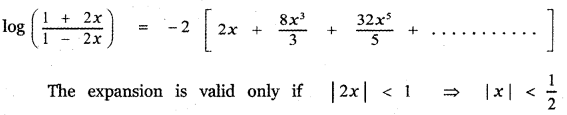Question 7.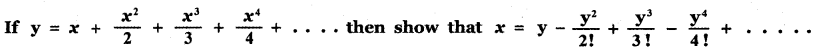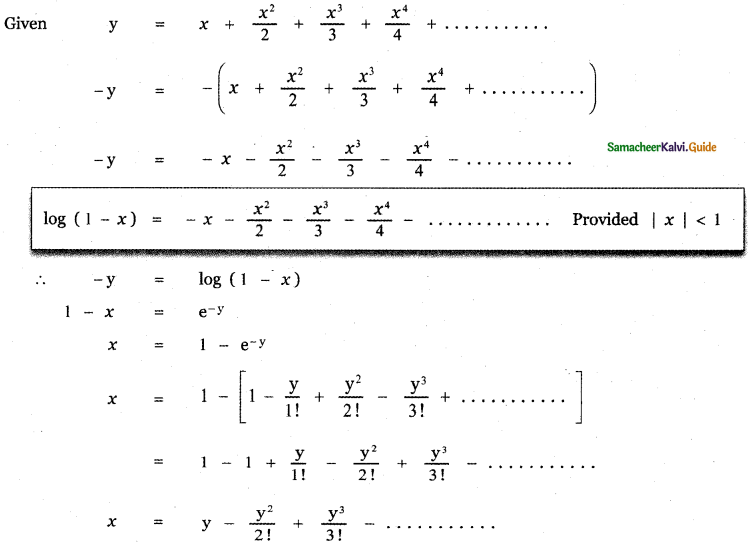Question 8.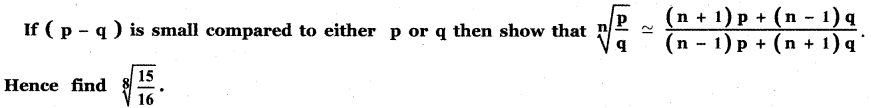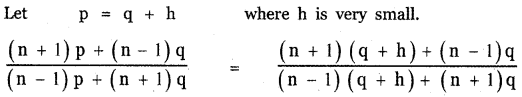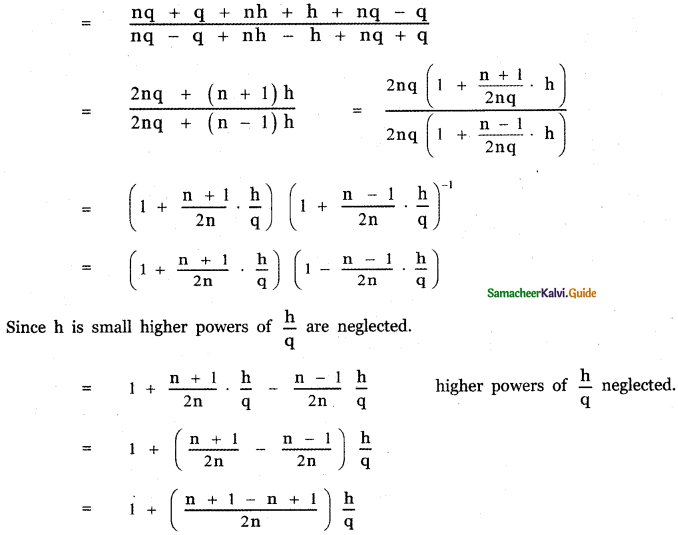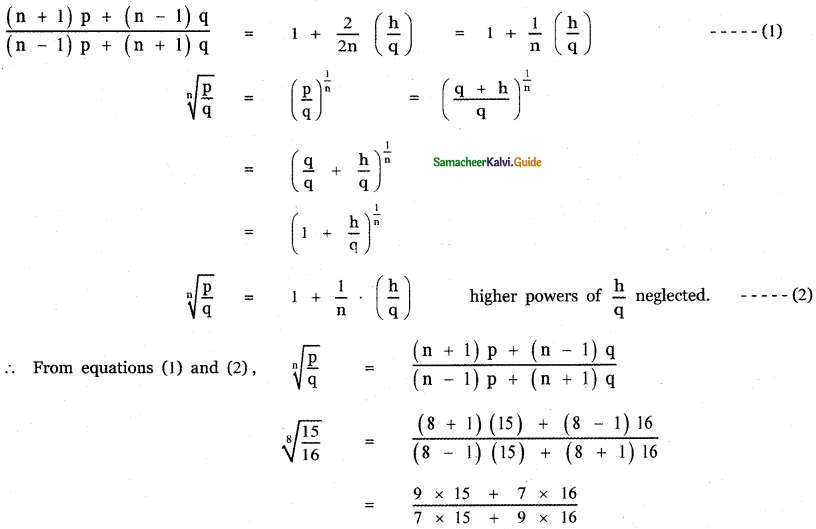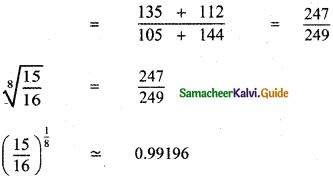Question 9.
Find the coefficient of x4 in the expansion of $$\frac{3-4 x+x^{2}}{e^{2 x}}$$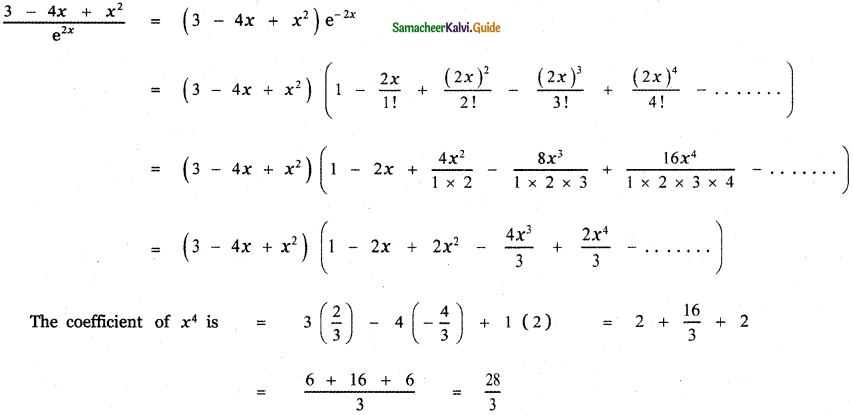Find the value of